Get instant live expert help with Excel or Google Sheets“My Excelchat expert helped me in less than 20 minutes, saving me what would have been 5 hours of work!”

#### Post your problem and you’ll get expert help in seconds.

Your message must be at least 40 characters
Our professional experts are available now. Your privacy is guaranteed.

# How to Subtract in Google Sheet and Excel

Excel does not have a subtract function. However, in this article, we have detailed foolproof ways of subtracting numbers, dates, time, percentages and matrices in excel and google sheets. These easy steps can be executed by all levels of Excel users from novice to expert.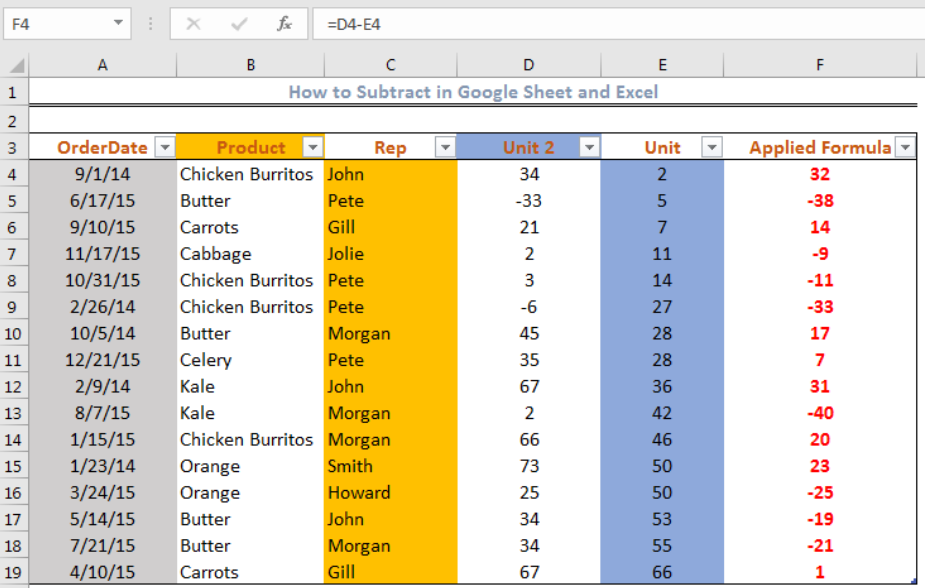Figure 1 – Applying the subtract function in Excel

## Using Subtraction Formula without Subtract Function

Excel does not have a subtract function. The minus sign (-) is the simplest function used.  Therefore, we will use the formula and steps below:

• In Cell F4 where we want the result to appear, we will type the equality sign, the first number, followed by the minus sign, and then, the second number

Formula: `= Number 1-Number 2`

Example: `=34-2`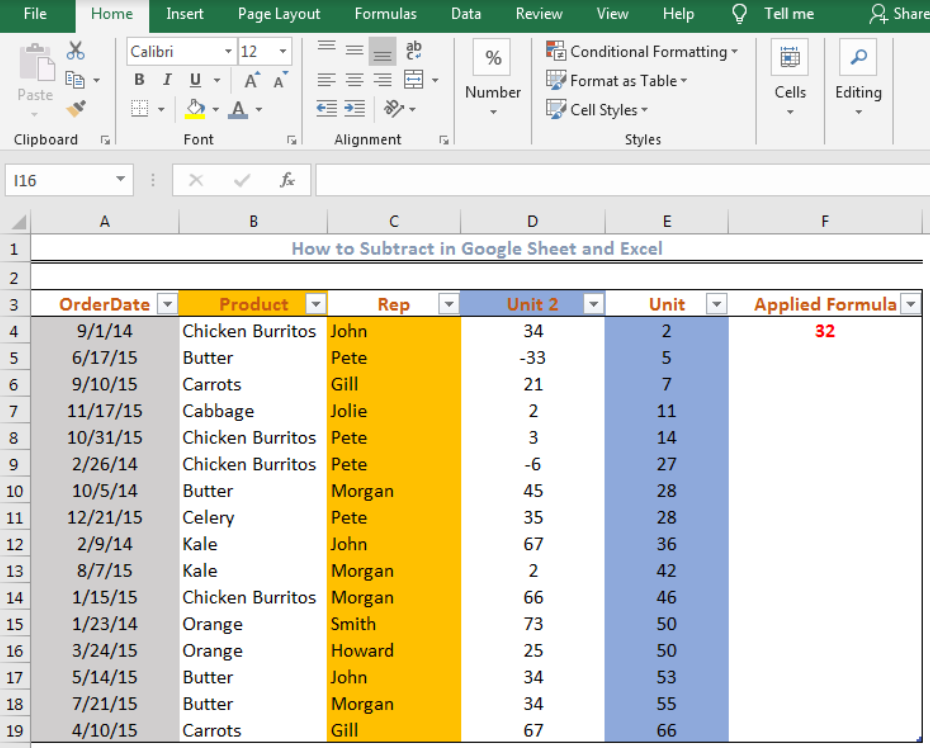Figure 2 – Subtracting two numbers in excel with the minus sign

It is important for us to know that this is a tedious procedure since we cannot automatically copy down the formula from Cell F4 to Cell F19. We will need to input the values manually.

## How to Subtract Cells in Excel

We can use the minus sign to subtract the value of one cell from another cell in excel. We will follow these steps:

• We will click Cell F4 where we wish to display our result.
• Next, we will enter the formula below into Cell F4 and PRESS ENTER.

`=D4-E4`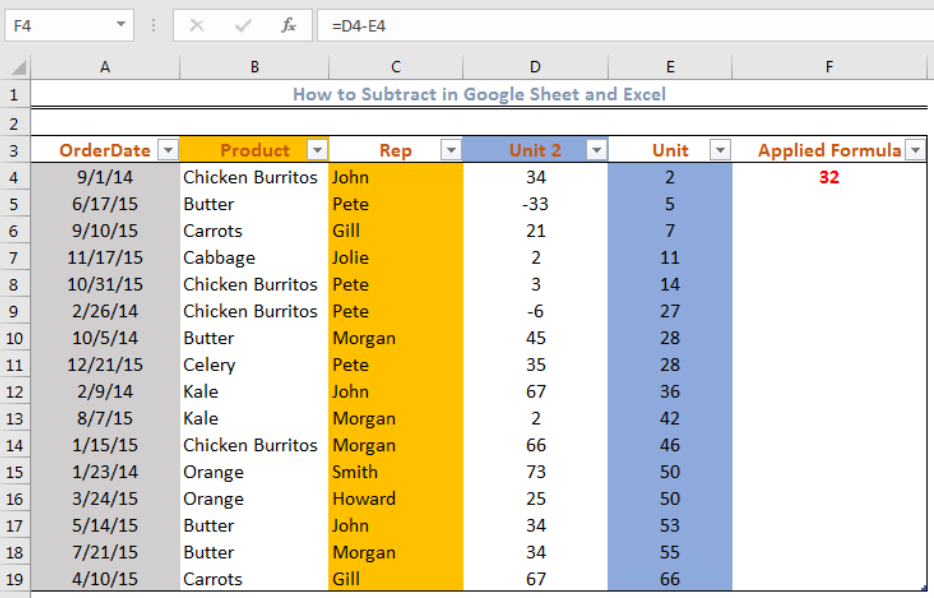Figure 3 – Subtract cells in Excel

Now, we can get the result for the other cells in Column F automatically by clicking Cell F4. We will then double-click on the small box at the bottom-right of Cell F4 and drag down to Cell F19.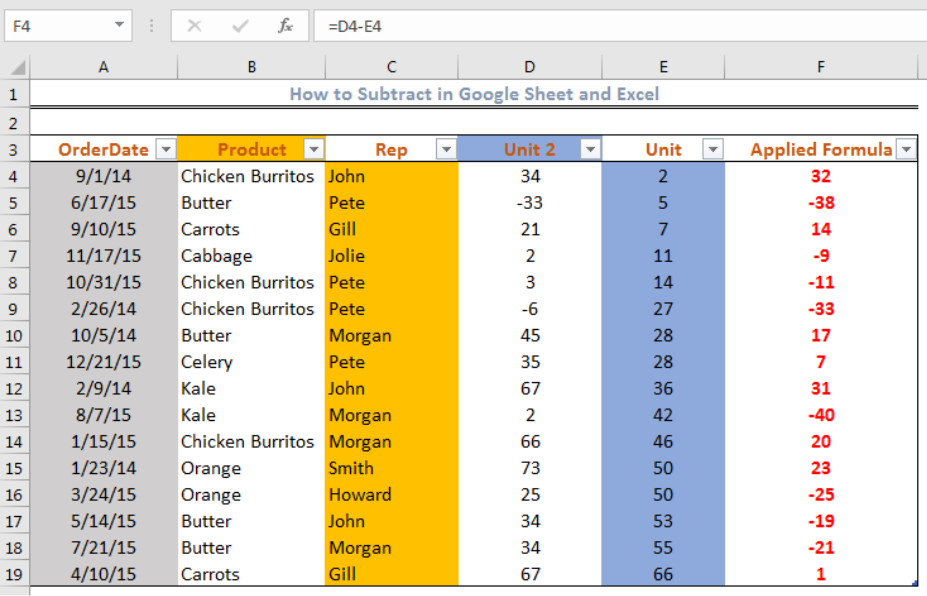Figure 4 – Subtract cells in Excel

## How to Subtract Multiple Cells from One Cell in Excel Using Minus Sign

• We will click on Cell F4, type the equality sign and click on the cells we wish to subtract from Cell D3. We will separate all the cells with a minus sign as shown below.

`=D4-E5-D6-E7-D8`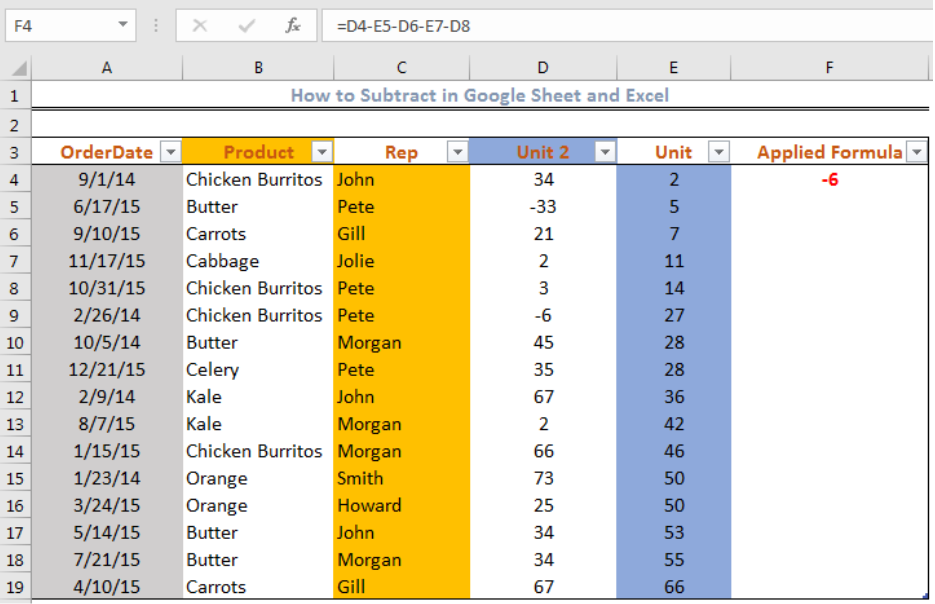Figure 5 – Subtract multiple cells from one cell using a minus sign

## Subtract Multiple Cells from one cell using the SUM function

With this formula, we will add the values in range E5 to E7 and subtract the result from Cell D4. We will click on Cell F4 and insert the formula: =D4-SUM(E5:E7). We can get the result for the other cells in Column F automatically by clicking Cell F4. We will then double-click on the small box at the bottom-right of Cell F4 and drag down to Cell F19.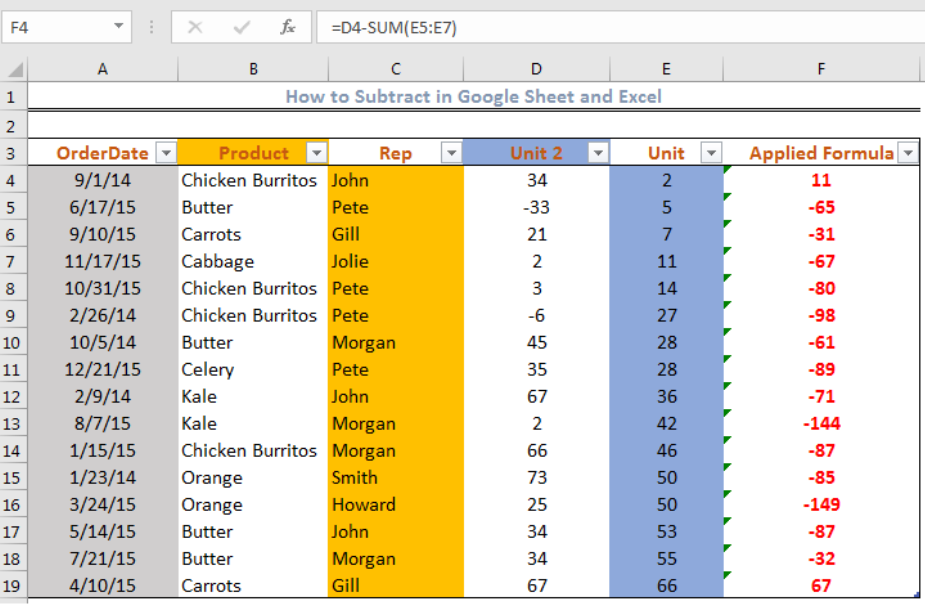Figure 6 – Subtracting multiple cells in Excel using the SUM function

## How to Subtract Columns in Excel

• To subtract columns row by row, we will click Cell F4 and insert the formula: =D4-E4
• We will drag the fill handle tool (the small box at the bottom-right when you click Cell F4) to enter the formula into the entire column.

`=D4-E4`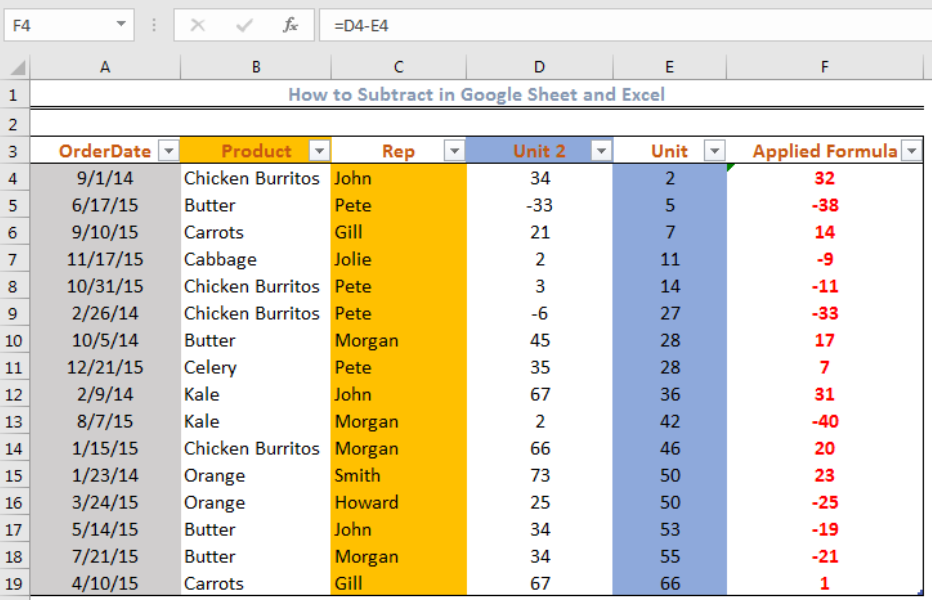Figure 7 – Subtract Columns in Excel

## Subtracting the same number from a column of numbers

We can subtract a number from numbers in a column. The number we will subtract is an absolute reference with the dollar sign so we can copy down the formula. We will use Cell E4 as the absolute cell reference. This cell does not change no matter where the formula is copied. We will insert the formula into Cell F4 and copy down after pressing enter.

`=D4-\$E\$4`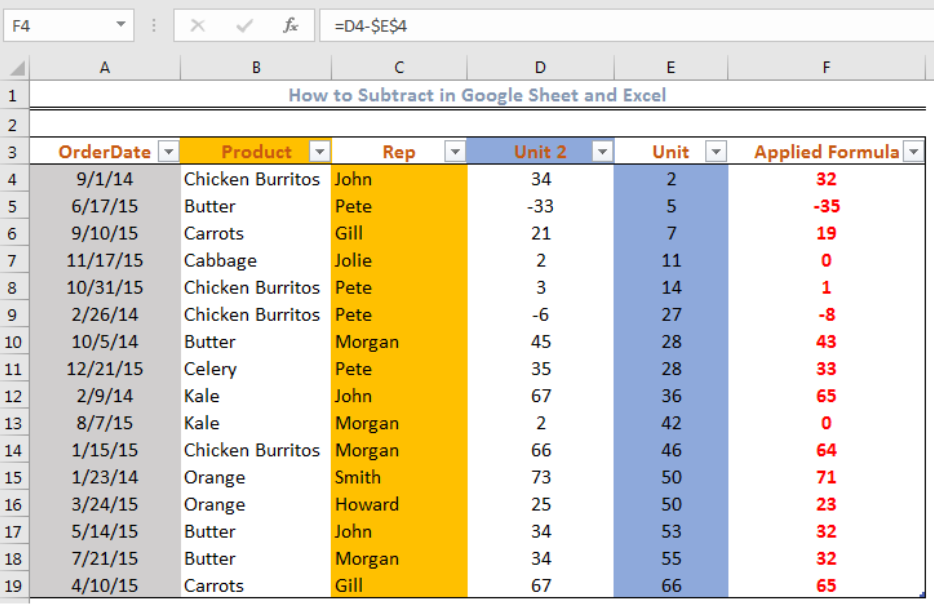Figure 8 – Using Cell E4 as an absolute cell reference

## How to Subtract percentages in Excel with minus formula

We can use cell references to subtract percentages with the minus sign. We will insert the formula into Cell F4 and press enter. We will drag the formula down the column to get the result of the other cells.

`=D4-E4`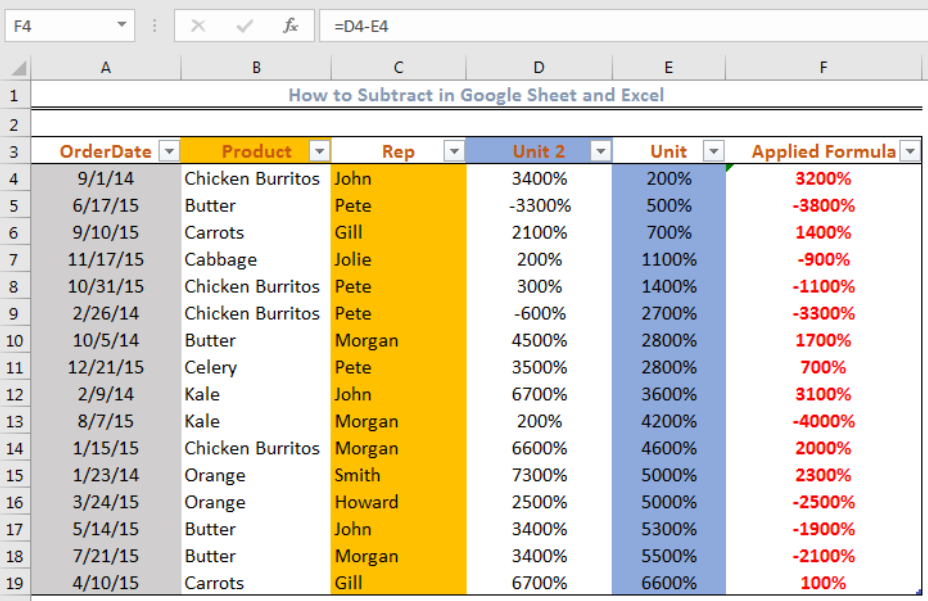Figure 9 – Subtract percentage using minus formula

## Subtract dates in Excel using Cell Reference and DATEVALUE function

We can subtract dates in excel using cell references with the formula below.

`=End_date-Start_date`

For instance, we can subtract the dates in Cell A4 and Cell B4 with the formula:  =A4-B4. We will click on Cell C4, insert the formula and press enter.Figure 10 – Subtracting dates with Cell reference

We can get the result for the other cells in Column C automatically by clicking Cell C4. We will then double-click on the small box at the bottom-right of Cell C4 and drag down to Cell C23.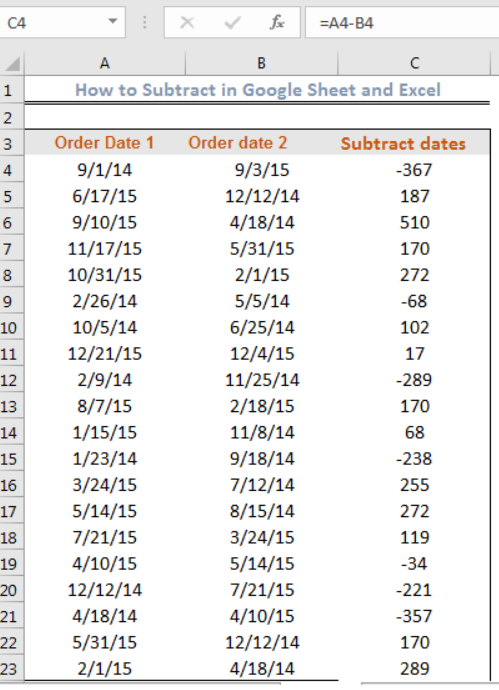Figure 11 – Subtracting dates with Cell reference

• We can also use the DATEVALUE function as displayed below. To display correctly, we must ensure the dates are in time format.

`=DATE(2014,9,1)-DATE(2015,9,3)`

• We will click on Cell C4, insert the formula below and press enter.

`=DATEVALUE("9/1/2014")-DATEVALUE("9/3/2015")`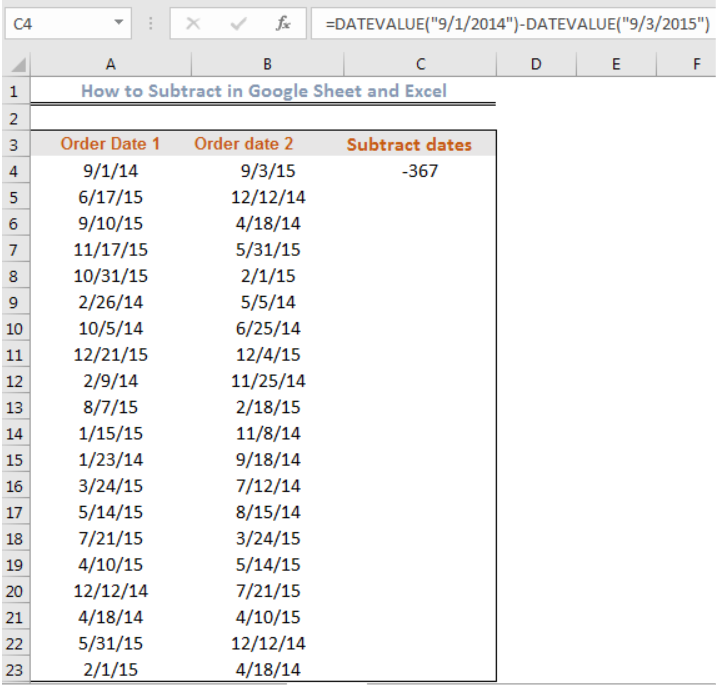Figure 12- Subtracting dates in Excel Using the DATEVALUE function

Unlike when we used cell reference, we cannot automatically get the result for the other cells with the DATEVALUE function.

## How to subtract time in Excel with Cell Reference

This follows a similar approach to subtracting dates in excel. We can subtract time in excel using cell references with the formula below.

`=End_time-Start_time`

We will use the formula below:

Example:` =A4-B4`

• Or we can use the TIMEVALUE function

`TIMEVALUE("4:30 PM")-TIMEVALUE("6:20 PM")`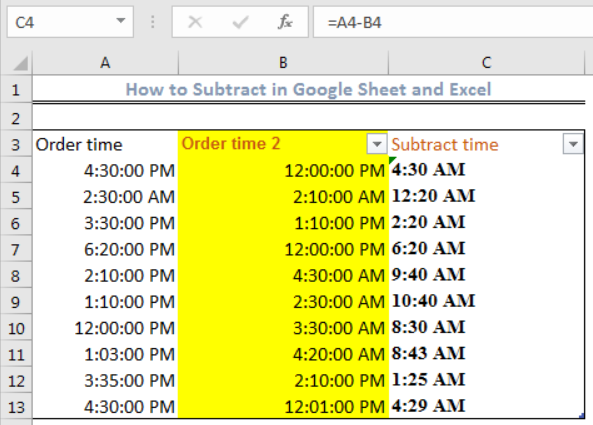Figure 13 – Subtracting time in Excel with Cell References

Note: We must write the time in the format as shown, leaving a space before “PM” or “AM.” Also, DATES and TIME are stored as numbers in excel, therefore, once our formula returns a number, we can convert it to time by right-clicking the cell, click on format cells and select time.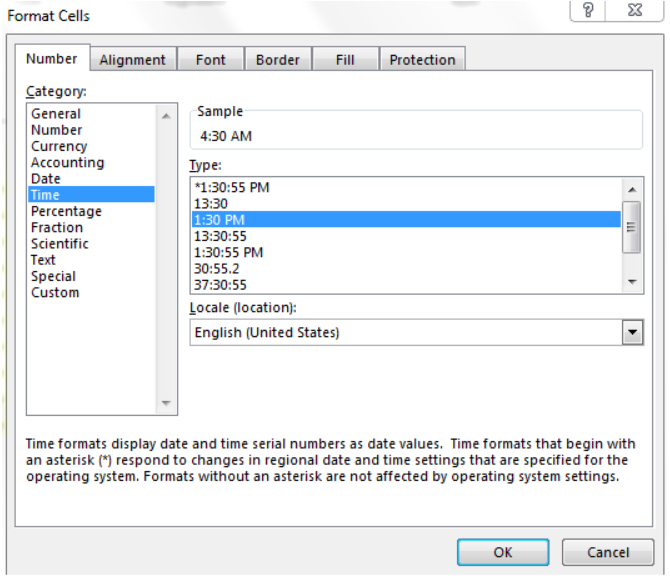Figure 14 – Format Cells Dialog box

## How to Subtract Matrices in Excel

We may have two sets of values (matrices) and want to subtract one set from the other. To do this, we can apply the matrix subtraction formula followed by Ctrl + Shift + Enter, which makes the formula an array formula.

For instance, we can subtract a matrix with the formula below:

`=(D4:E4)-(D5:E6)`

We will insert this formula in Cell F4 and press Ctrl + Shift + Enter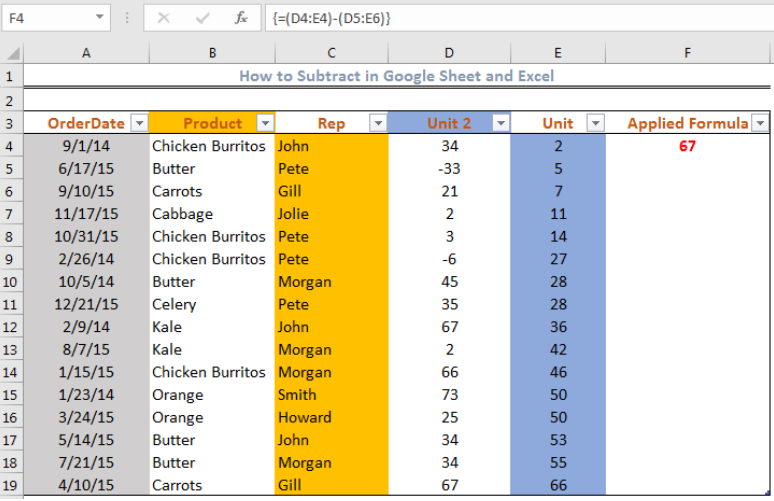Figure 15– Subtracting matrices

## Instant Connection to an Excel Expert

Most of the time, the problem you will need to solve will be more complex than a simple application of a formula or function. If you want to save hours of research and frustration, try our live Excelchat service! Our Excel Experts are available 24/7 to answer any Excel question you may have. We guarantee a connection within 30 seconds and a customized solution within 20 minutes.

Solution examplesWhat is the formula to add 4 cell and add them together in another cell then in another call get the average of that cell
Solved by C. J. in 37 minsI need a formula to pop at the upper left corner of a spreadsheet. If I enter the month "January," I want the column number sum of January =SUM(AB11:AB75) from another section on the same excel page to pop right below the "January" cell, and not display the formula expression, but see the \$100.
Solved by T. Q. in 40 minsCan't add (SUM) in imported numbers from bank account
Solved by F. C. in 40 minsI need a formula to combine D2 to D100 to add together a column of numbers, then take away the same amount on the same row when column E is filled. i.e. column D is a price of an item, so the formula must calculate the total, then when the item is sold an 'a' is marked next to the item in column E, the formula then must deduct this amount from the total
Solved by X. W. in 20 minsI would like to have a diagram in a new sheet, where the horizontal axis is the days, as they are in column DX. Each day shall show the sum of all unique leads of that day, and I would like to be able to check via a box of checkboxes, which facilities are shown, the facilities are in column BC.
Solved by I. A. in 45 mins